## Introduction

Topological phases of matter escape the canonical characterization of states dictated by the Ginzburg–Landau theory of phase transitions. These phases emerge without breaking symmetries and are not characterized by a long-range order nor a local order parameter but rather by a global topological order. Historically, topology was first proven to have a key role in explaining algebraically decaying order, transport and coherence of two-dimensional Bose liquids, XY models and crystals1. Shortly after, the quantization of Hall conductance2 was shown to be rooted in current-carrying edge states, protected by the topology of the bulk3,4,5. Being associated with a global order, these phases are robust against local perturbations and promise important applications in metrology, spintronics and quantum computation (see, for example, refs 6, 7, 8).

Intense studies9 followed the early discoveries, and topological insulators have by now been engineered in a variety of physical architectures, such as superconducting10, mechanical11, optomechanical12, photonic13, atomic14 and acoustic platforms15. Such diverse systems have been exposed to either real or synthetic magnetic fields, and their topological properties have been studied by scattering at the interface between different domains15,16 or imaging edge states17,18,19,20,21,22,23,24,25,26. Direct detection of topological invariants in the bulk of the system (with no need of edges) has been reported so far by very few experiments27,28,29.

Topological insulators are classified in terms of dimensionality and discrete symmetries30. One-dimensional (1D) systems with chiral symmetry are characterized by the Zak phase, that is, the Berry phase accumulated by an eigenstate during its parallel transport through the whole Brillouin zone31. The Zak phase is closely related to the electric polarization in solids and plays a key role in the modern theory of insulators32,33.

Periodically driven (Floquet) systems are attracting an increasing interest, as these show richer topological features than their static counterparts17,24,25,26,34,35,36,37,38,39,40,41,42,43,44. Particularly promising Floquet topological systems are discrete-time quantum walks (QWs)16,17,29,45,46,47, and recent works have reported the observation of topological invariants16,29, quantum phase transitions46 and edge states17 in these systems. In its simplest version, a QW is the discrete time evolution of a particle (the walker) on a 1D lattice48. At each step, the walker moves to neighbouring sites, with the direction of the shift depending on the state of an internal two-level degree of freedom (the coin). Between consecutive steps, a rotation modifies the coin state, univoquely determining the following evolution.

Here we demonstrate that, in chiral 1D static and Floquet systems with spin 1/2 (that is, a two-state coin), the mean chiral displacement of a particle’s wavepacket becomes quantized and proportional to the Zak phase in the long-time limit. Remarkably, this occurs during the free evolution of the system, in absence of any external force or loss mechanism, with the only requirement that the initial wavefunction is localized. We validate experimentally this finding in a photonic discrete-time QW based on the orbital angular momentum (OAM) of a light beam. We implement the same QW in a shifted inequivalent timeframe and measure a second Zak phase. Combining the two windings, we extract the complete set of topological invariants characterizing the system. Finally, we prove the robustness of our detection by adding dynamical disorder. These measurements provide therefore a bulk measurement of the Zak phases and complete topological invariants of a 1D chiral QW. Our proposal may be straightforwardly applied to general driven Floquet systems.

## Results

### Zak phase detection in the bulk of a QW

In one dimension, discrete-time QWs with chiral symmetry display a quantized Zak phase and have been extensively studied in the past years. Among these implementations, we focus on the photonic platform proposed in ref. 46. Here the walk takes place on a lattice whose sites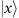are associated with photonics states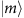, corresponding to light beams that carry units of OAM per photon along the propagation axis and show a twisted wavefront49. The two coin states are instead mapped onto the left and right circular polarizations of the beam, carrying ±ħ units of spin angular momentum per photon along the propagation axis. Once the system is prepared in an initial state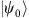, its state after t timesteps is given by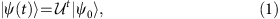where the single-step operator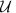is obtained by cascading suitable combinations of quarter-wave plates and q-plates46,50,51. In Fig. 1a, we show a pictorial representation of our setup that realizes a seven-step QW withimplemented specifically as U ≡ Q·W46. The action of a quarter-wave plate oriented at 90° with respect to the horizontal direction is described by the local operator W, rotating the polarization states as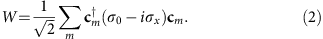Here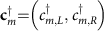creates a particle on site m with polarization L/R and σi are Pauli matrices acting in the coin (polarization) space. The translation operator Q is implemented by a q-plate, a liquid crystal device that yields an effective spin–orbit interaction in the light beam. This couples neighbouring sites and polarization states as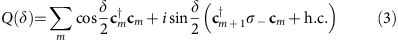where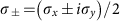are the operators that flip the coin statesand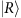, δ is the optical retardation of the q-plate and h.c. stands for Hermitian conjugate. Further details on the q-plates and on the complete experimental setup are provided in the Methods section and Supplementary Fig. 1.

Very generally, QWs are generated by the repeated application of a unitary operator, and therefore the system can be described in the framework of Floquet theory. As a consequence of translational invariance in space, the effective Hamiltonian associated with a full period is diagonal in momentum space and may be written as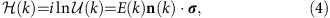with E(k) the quasi-energy dispersion,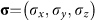and we have set the period T and ħ to unity. The point on the Bloch sphere identified by the unit vector n(k) represents the coin part of the system eigenstates, while their spatial part is a plane wave with quasi-momentum k46. The function ln(x) denotes the principal branch of the natural (matrix) logarithm, so that the quasi-energy is a periodic function, with −π and +π identified.

The class of QWs we are considering features chiral symmetry, since there exists a unitary operator Γ such that Γ2=I and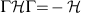. These conditions imply that Γ is Hermitian and that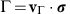, with vΓ a vector labelling a point on the Bloch sphere. In this case, the unit vector n is bound to rotate around the origin in a plane orthogonal to vΓ, and the Zak phase equals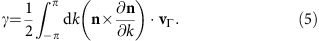The winding number γ/π assumes strictly integer values and counts the number of times the unit vector n rotates around the unit vector vΓ as k traverses the whole Brillouin zone. In Fig. 1b, we show the winding of the vector n of the operator U, for two values of δ in different topological sectors. The Zak phase is therefore a bulk property; although it has strong influences in properties of systems where it arises, its detection in current experimental architectures remains challenging.

In the following, we show that information on such topological invariant is hidden in the subleading terms of the mean displacement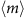, when the initial wavepacket is localized on a single site. This extends a previous result showing that, in the same conditions, the ballistic terms of higher moments of the walker’s displacement feature discontinuities at topological phase transitions46. Let us consider the evolution of a wavepacketinitially localized at site m=0, and whose polarization is characterized by the expectation values of the three Pauli matrices,. The mean displacement of the wavepacket after t timesteps is given by (see Supplementary Note 1 for details)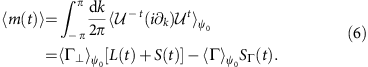The term in square brackets in equation (6) is proportional to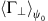, the projection of the initial polarization on a direction orthogonal to vΓ, and contains a ballistic term L(t) (which grows linearly with t) and a subleading part S(t).

The vector identifying the specific direction of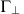in the plane orthogonal to vΓ, and the explicit functional forms of L(t) and S(t), are non-universal features that depend on the specific protocol (or timeframe) and have no particular relevance for our discussion. The second term in equation (6), which is weighted by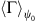(the projection of the initial polarization along vΓ), is the subleading chiral term SΓ that may be written as (see Supplementary Note 1 for details)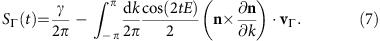In the limit t→∞, SΓ becomes proportional to the Zak phase, as the oscillatory correction quickly averages to zero (see Fig. 1c).

The above analysis shows that information on the Zak phase is contained in the mean displacement of the walker, and it may be extracted by fittingat long times, isolating in turn the second term of equation (6). A related result for the case of a non-Hermitian QW initialized on a chiral eigenstate (that is, an initial condition such that) was demonstrated theoretically in ref. 52 and verified experimentally in ref. 29. However, this measurement would not be robust. Indeed, even if one prepared the initial polarization in an eigenstate of the chiral operator Γ, so that=0, disorder during the propagation of the beam would introduce polarization components orthogonal to vΓ. These would give rise to ballistic contributions, which in the long-time limit would dramatically affect the result.

An alternative and more convenient approach consists in measuring the mean chiral displacement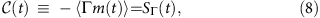which quantifies the relative shift between the two projections of the state onto the eigenstates of the chiral operator (see Supplementary Note 1 for a concise derivation of this equality). Importantly, the result contained in equation (8) is (i) independent of the initial polarization and (ii) robust against disorder. We probe the chiral displacement in our photonic platform by performing a seven-step QW of the protocol U=Q·W, as depicted in Fig. 1a. The chiral eigenstates correspond to two specific orthogonal polarization states, which depend explicitly on the protocol, and which we detect at the end of the QW (see Methods section). In Fig. 1c, we report the measured values of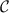for two different initial polarization states. Experimental points closely follow the theory curve for seven time steps (blue solid line), and no significant differences can be observed between the two different initial states, proving that this measurement is insensitive to the choice of the polarization of the photons. For completeness, we also show results predicted for 33 steps, and the asymptotic long-time limit, which coincides with the Zak phase (over 2π). We note here that, although both theory and data oscillate, as few as seven steps are enough to have a clear detection of the Zak phase.

### Zak phase in a shifted timeframe

In static systems, bulk topological invariants such as the Zak phase or the Chern number are uniquely defined by integrals over the whole Brillouin zone and are in one-to-one correspondence with the presence of edge states, thus providing a full classification in terms of the periodic table of topological insulators30. The situation is very different in periodically driven (Floquet) systems in D dimensions, where the integral determining the topological invariants needs to be performed over a D+1 dimensional torus constituted by the Brillouin zone and an extra periodic dimension, the quasi-energy42.

Moreover, a gauge freedom is introduced by the choice of the timeframe, that is, the origin of time of the periodic cycle (see Fig. 2a). While the dispersion E(k) is equal in all timeframes, the effective Hamiltonian, its eigenstates and symmetries and the resulting dynamics crucially depend on the timeframe40. As an example, the operator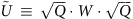defines a timeframe that is inequivalent to the one introduced by U. In particular, the unit vector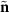(k) defined by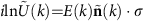may wind twice around the chiral axis as k traverses the Brillouin zone (see Fig. 2c), and its Zak phase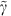(dashed line in Fig. 2d) differs from the Zak phase γ of protocol U (dashed line in Fig. 1c).

We realize experimentally protocol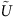by the setup shown schematically in Fig. 2b. Using the relation, it is straightforward to see that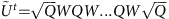. Hence, we realize the operator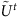by placing q-plates yielding an optical retardation δ/2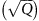at the beginning and end of the optical path, while in the bulk of the walk we adopt the same sequence reported in Fig. 1a (with the last q-plate removed). Overall, our QW implements seven steps of protocolby means of a total of eight q-plates, six with retardation δ and two tuned at δ/2 (first and last plates), separated by quarter-wave plates. In Fig. 2d, we report the measure of the mean chiral displacement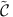generated by the single-step operator. As in the case of protocol U, this quantity accurately follows the theory prediction, providing an unambiguous detection of the Zak phaseof the infinite system after just seven steps.

### Complete topological characterization

It is clear from the previous discussion that the Zak phase associated with a single timeframe does not contain all the topological information of our QW. Indeed in Floquet 1D chiral systems, there exist two independent classes of protected edge states at either 0- and π energies. An example of these edge states is shown in Fig. 3a, where we plot the quasi-energies of all eigenstates of an open-ended lattice. As remarked above, the spectrum is independent of the timeframe. The spectrum contains edge states even for 3π/2<δ<5π/2 where the Zak phase γ of protocol U is zero, explicitly confirming that the Zak phase of a single QW protocol does not contain the complete information about the topological state of the system.

The bulk-edge correspondence in these driven systems requires two invariants C0 and Cπ, yielding, respectively, the number of 0- and π-energy edge states. As shown in refs 38, 41, these are simple functions of two Zak phases, measured in two inequivalent timeframes possessing an ‘inversion point’, that is, which may be written, respectively, as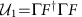and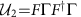, with F a suitable evolution operator. In the case of our setup, the two special protocols fulfilling this criterion areand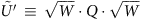. However, it is simple to show that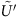is topologically equivalent to U, as no gap closing happens during the rotation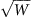; therefore the Zak phase ofcoincides with γ. As such, the complete topological classification of 1D chiral systems may be obtained by means of the two quantities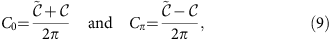which converge in the long-time limit, respectively, to the number of 0- and π-energy edge states. By combining our measurements of the mean chiral displacements measured in the inequivalent timeframes, we are now able to compute the invariants C0 and Cπ and detect the complete phase diagram of this system: the result is shown in Fig. 3b. Once again, our measurements show a remarkably fast convergence towards the asymptotic limit.

### Robustness to dynamical disorder

Finally, we test the stability of the quantization of the mean chiral displacement against disorder. In particular, we choose protocol U and introduce dynamical disorder by offsetting the optical retardation δj (1≤j≤7) of each q-plate by a small random amount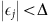around their common mean value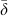. In our experiment, we set Δ=π/10 and π/5. We note that this disorder is dynamic, in the sense that it affects independently the various q-plates crossed by the beam, but crucially it respects chiral symmetry. This can be simply understood by noting that the vector vΓ, defining the chiral operator, does not depend on δ.

As shown in Fig. 4, in single realizations the mean chiral displacement presents oscillations featuring higher amplitude for increasing disorder, but an ensemble average over independent realizations smoothly converges to the expected theoretical result, which in the infinite time limit gives the bulk value of the Zak phase. Here we performed measurements on protocol U, but similar robustness of the chiral displacement shall hold for every 1D QW chiral protocol, and more generally every 1D chiral system, as long of course as the disorder does not break chiral symmetry and its strength is small compared to the gap size to prevent interband transitions. As an example, in the Supplementary Note 2 and Supplementary Figs 2–5 we show that the mean chiral displacement is an equally robust topological marker for a completely different and static (that is, not driven) system, the celebrated SSH model.

## Discussion

Summarizing, here we proposed an efficient method to measure the Zak phase of a chiral system by direct observation of its free bulk dynamics. In particular, we showed that information on the topological phase of the bulk is encoded in the mean chiral displacement, an oscillatory quantity that rapidly converges to the Zak phase, and is robust against (chiral-preserving) disorder in both space and time.

We experimentally verified our findings by performing the first measurement of the Zak phase of a chiral QW. The physical platform we chose is a photonic setup based on the OAM of a light beam, where the mean chiral displacement corresponds to the relative shift of the two chiral polarization components. A precise readout of the Zak phase was obtained after only seven QW steps. We further used the same method to measure the Zak phase in a complementary timeframe, which we realized by swapping few optical components. By combining the two measurements, we extracted the two invariants providing the complete bulk-edge correspondence for this driven system, that is, the one associated to the 0-energy edge state, and the one connected to the anomalous π-energy edge state. Finally, we proved that the mean chiral displacement is a robust measure of the Zak phase by introducing dynamical but chiral-preserving disorder.

Although here we investigated experimentally a specific QW, our results are not restricted to QWs nor to Floquet systems. Indeed, the mean chiral displacement provides a robust topological characterization of arbitrary spin-1/2 1D chiral systems, either static or periodically driven. These may nowadays be realized in a variety of platforms, ranging from ultracold atoms in optical lattices to photonic waveguides and from semiconductor quantum wells to optomechanical systems.

While formerly known methods for detection of topological properties require a uniform filling of the band of interest, external forces, loss mechanisms or fine-tuning so that only edge states are populated, the method proposed here quite remarkably achieves this goal by observing the free evolution of a single particle, initially localized on a single site in the bulk. This aspect may be specially beneficial for systems where filling a band is intrinsically challenging, such as bosonic condensates or phononic and photonic ensembles.

Future interesting directions opened by this work include the extension of our results to chiral systems with more than two internal states, a further understanding of the role played by temporal disorder and the topological characterization of systems in higher spatial dimensions.

## Methods

### Experimental setup

Our apparatus is shown schematically in Figs 1a and 2b, and a more detailed description is given in Supplementary Fig. 1. We produce a TEM00 mode by coupling the output of a Ti:Sa laser (λ=800 nm) to a single-mode fibre, thus preparing the beam in an OAM state with m=0. At the exit of the fibre, a specific polarization is selected by means of a sequence of a quarter-wave plate and a half-wave plate. Therefore, the initial state of the QW is=, where m is the position in the walker (OAM) and space s its coin state (polarization). In the standard protocol U=Q·W, the single step consists of a quarter-wave plate oriented at 90° with respect to the horizontal direction (operator W), followed by a q-plate (operator Q), as shown in Fig. 1a. To implement the second protocol, we exploited the fact that equation (3) may be written as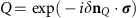, that is, it corresponds to a rotation around a suitable unit vector nQ; as such, a q-plate with retardation δ/2 implements the desired operator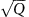. To implement the single-step operator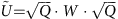, we then added a δ/2 q-plate at the beginning of the sequence, and we halved the retardation of the last q-plate, as shown in Fig. 2b.

### q-plates

Each q-plate consists of a thin layer of birefringent liquid crystals, whose optic axes are arranged in a singular pattern characterized by a topological charge q (in our case, q=1/2). The patterned birefringence gives rise to an optical spin–orbit coupling that induces the polarization-dependent shift of OAM. Along with the specific pattern, the action of each device is determined by its optical retardation δ, as reported in equation (3). The optical retardation can be continuously tuned by applying an electric field, allowing in turn for an accurate control of the spin–orbit interaction53.

### Detection of the chiral displacement

At the end of the walk, we can select any polarization component of the final state by a combination of a quarter-wave and a half-wave plate, followed by a linear polarizer, and we measure its OAM content by diffraction on a spatial light modulator coupled to a single-mode fibre and a power meter, which records the light intensity. Since we are interested in analysing the OAM spectrum of chiral components of the final wavepacket, waveplates orientations are selected so as to implement polarization projections onto chiral states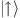and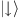. The chiral operators for protocols U andare, respectively,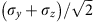and σz, so it is straightforward to see that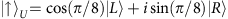and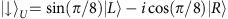for protocol U, while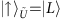and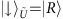for protocol. The combination of polarization and OAM projections allows for determining the probabilities Pi,m, with i={↑, ↓}, that the system is in the chiral state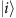and in the OAM state. Given the probability distributions Pi,m, the chiral displacement is simply given by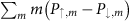.

### Data availability

The complete set of raw data supporting the findings of this study is available from the corresponding authors upon request.